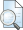# The Effect of Air Flow Velocity In Coping Wood Gasification Toward Combustion Temperature and Effective Combustion Time

Anindito, Dhimas Cahyo (2015) The Effect of Air Flow Velocity In Coping Wood Gasification Toward Combustion Temperature and Effective Combustion Time. Skripsi thesis, Universitas Muhammadiyah Surakarta.PDF (Bab 1) CHAPTER I.pdf Download (79kB)PDF (Bab 2) CHAPTER II.pdf Restricted to Repository staff only Download (110kB)PDF (Bab 3) CHAPTER III.pdf Restricted to Repository staff only Download (190kB)PDF (Bab 4) CHAPTER IV.pdf Restricted to Repository staff only Download (149kB)PDF (Bab 5) CHAPTER V.pdf Restricted to Repository staff only Download (62kB)PDF (Naskah Publikasi) PAPER.pdf Download (274kB)PDF (Daftar Pustaka) REFERENCES.pdf Download (63kB)PDF (Halaman Depan) HALAMAN DEPAN.pdf Download (253kB)PDF (Lampiran) APPENDIX.pdf Restricted to Repository staff only Download (85kB)PDF (Pernyataan) SURAT PERNYATAAN PUBLIKASI KARYA ILMIAH.pdf Restricted to Repository staff only Download (42kB)

## Abstract

Nowadays, coping wood is one of the most reliable solutions for alternative energy. Coping wood can produce methane by using gasification technology. By those facts, this research has been held to find out the effect of air flow velocity toward combustion temperature in lumber gasification. The first step of this method applied four variation of air flow velocities such as v=7.6 m/s, v=6.9 m/s, v= 5.6 m/s, and v=4.2m/s. Temperature measurements were recorded every minute, and by the height and deepness of fire. Thermocouple has been put at the centre-bottom of fire for recording temperature changing in every minute. By the height of fire temperature measurement, the thermocouple put at the outer bottom, middle, and top of fire. The result of experiment which measured the highest temperature from each air flow velocity variation refers at v=7.6 m/s is 5710C, at v=6.9 m/s is 6000C, at v=5.6 m/s is 6200C, and at v=4.2m/s is 6090C. Whereas the temperature measurement based on the height of fire show results such as, at v=7.6 m/s, T1=4720C, T2=4400C, T3=4300C. At v=6.9 m/s, the results are T1=4700C, T2=4700C, T3=4000C. At v=5.6 m/s, the results are T1=4500C, T2=4720C, T3=4440C. And at v=4.2 m/s, the results are T1=4800C, T2=4600C, T3=4000C. For the temperature measurement based on the deepness of fire, the result can be shown as t1=4670C, t2= 5100C, t3=5200C at v=7.6 m/s. at v=6.9 m/s, the results are t1=4690C, t2=5300C, t3=5800C. The temperature result at v=5.6 are t1=4510C, t2=5000C, t3=5600C. And for v=4.2 m/s, the results are t1=4720C, t2=4990C, t3= 5700C. Total time of gasification at v=7.6 m/s is 780 s, v=6.9 m/s is 720 s, v=5.6 m/s is 698 s, and v=4.2 m/s is 765 s

Item Type: Karya ilmiah (Skripsi) coping wood, gasification, air flow velocity, effective combustion time, combustion temperature. T Technology > TJ Mechanical engineering and machinery Fakultas Teknik > Teknik Otomotif Unnamed user with username d200112006 30 Dec 2015 04:54 30 Dec 2015 04:54 http://eprints.ums.ac.id/id/eprint/40084

### Actions (login required)View Item